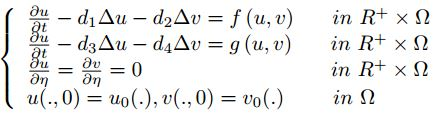• Abstract
• Keywords
• References
• PDF
• Abstract

The purpose of this paper is to prove the global existence in time of solutions for the strongly coupled reaction-diffusion system:with full matrix of diffusion coefficients. Our techniques of proof are based on Lyapunov functional methods and some $$L^{p}$$ estimates. we show that global solutions exist. Our investigation applied for a wide class of the nonlinear terms f and g.

• Keywords

Global Existence, Reaction Diffusion Systems, Lyapunov Functional.

• References

 N. Alikakos, LP bounds of solutions of reaction-diffusion equations, Comm. Partial Differential Equations 4 (1979), 827--828.

 H. Amann, Dynamic theory of quasilinear parabolic equations - I. Abstract evolution equations, Nonlinear Anal. 12 (1988), 895-919.

 S. Bonaved, D. Schmitt, Triangular reaction-diffusion systems with integrable initial data, Nonlinear. Anal 33 (1998), 785--801.

K. Boukerrioua,existence of global solutions for a system of reaction-diffusion equations having a full matrix Ser. Math. Inform. Vol. 29, No 1 (2014), 91--103

 T. Diagana, Some remarks on some strongly coupled reaction-diffusion equations, J.Reine. Angew., 2003.

 R. Fisher, The advance of advantageous genes, Ann. Eugenics 7 (1937), 335--369.

 H. Fujita, On the blowing up of solutions to the Cauchy problem for ∂u/∂t=Δu+u^{^{(σ+1)}} J. Fac. Sci. Univ. Tokyo Sect. A Math. 16 (1966), 105--113.

 A. Haraux, M. Kirane, Estimation C1 pour des problèmes paraboliques semi-linéaires,Ann. Fac. Sci. Toulouse Math. 5 (1983), 265--280.

 A. Haraux, A. Youkana, On a result of K. Masuda concerning reaction-diffusion equations, Tohoku. Math. J. 40 (1988), 159--163.

 D. Henry, Geometric Theory of Semilinear Parabolic Equations, Lecture Notes in Math., 840, Springer Verlag, New York, 1981.

 S. L. Hollis, R. H. Martin, M. Pierre, Global existence and boundedness in reaction diffusion systems, SIAM. J. Math. Anal. 18(3) (1987), 744--761.

 J. I. Kanel and M. Kirane, Pointwise a priori bounds for a strongly coupled system of reaction-diffusion equations with a balance law, Math. Methods Appl. Sci. 21 (1998), 1227-1232.

 I. Kanel, M. Kirane, Global existence and large time behavior of positive solutions to a reaction diffusion system, Differ. Integral Equ. Appl. 13(1--3) (2000), 255--264.

 S. Kouachi, Global existence of solutions to reaction diffusion systems via a Lyapunov functional, Electron. J. Differential Equations (68) (2001), 1--10.

 S. Kouachi, Global existence of solutions for reaction-diffusion systems with a full matrix of diffusion coe cients and nonhomogeneous boundary conditions,Electron. J. Qual. Theory Di er. Equ. 4 (2002), pp. 1-10.

 S. Kouachi, Global existence of solutions in invariant regions for reaction-diffusion systems with a balance law and a full matrix of di usion coe cients,Electron. J. Qual. Theory Differ. Equ. 2 (2003), pp. 1-10.

 S. Kouachi, Invariant regions and global existence of solutions for reaction-diffusion systems with full matrix of diffusion coefficients and nonhomogeneous boundary conditions, Georgian Math. J. 11 (2004), 349-359.

 S. Kouachi, A. Youkana, Global existence and asymptotics for a class of reaction diffusion systems, Bull. Polish Acad. Sci. Math. 49(3), 2001.

 R. H. Martin, M. Pierre, Nonlinear reaction-diffusion systems, in: Nonlinear Equations in the Applied Sciences, Math. Sci. Eng. Acad. Press, New York 1991.

 K. Masuda, On the global existence and asymptotic behavior of solutions of reaction diffusion equations, Hokkaido Math. J. 12 (1983), 360--370.

 A. Moumeni, L. Salah Derradji, Global existence of solution for reaction diffusion systems, IAENG, Int. J. Appl. Math. 40(2) (2010), 84--90.

 A. Moumeni, L. Salah Derradji, Global existence of solution for reaction diffusion Systems with non diagonal matrix, Demonstratio Mathematica, Vol. XLV No 1( 2012 ).

 J. D. Murray, Mathematical Biologie, 3rd ed., Interdisciplinary Applied Mathematics,Springer Verlag, 2002.

 A. Pazy, Semigroups of linear operators and applications to partial differential equations,Applied Mathematical Siences, Springer--Verlag, New York, 1983.

 M. Pierre, D. Schmitt, Blow up in reaction-diffusion systems with dissipation of mass,SIAM. J. Math. Anal. 42(1) (2000), 93--106.

 B. Rebiai and S. Benachour, Global classical solutions for reactiondiffusion systems with nonlinearities of exponential growth, J. Evol. Equ.10 (2010), 511--527

 F. Roth, Global solutions of reaction diffusion systems, Lecture Notes in Math. 1072,Springer Verlag, Berlin, 1984.ViewDownloadArticle ID: 4683DOI: 10.14419/gjma.v3i3.4683